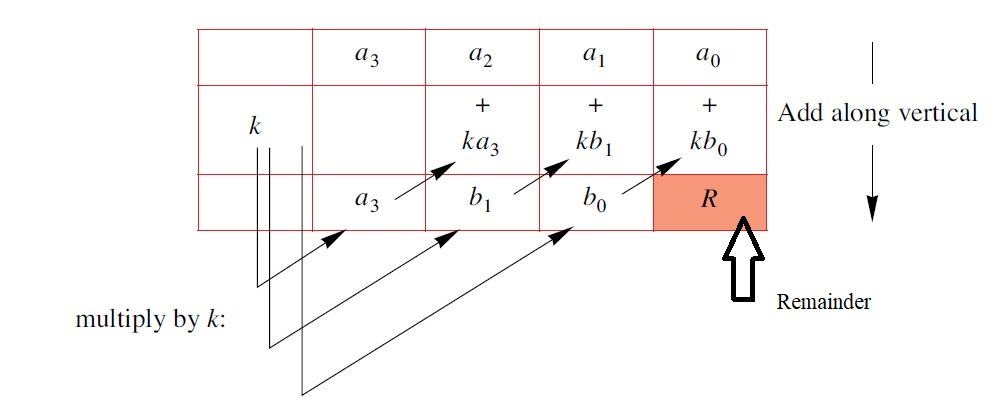# Notes – Polynomials

A polynomial function, $p\left(x\right)$ , is an algebraic expression that takes the form

where the coefficients  are real numbers, and the powers are non- negative integers

The degree of a polynomial is the highest power of  $x$ in the expression.

SYNTHETIC DIVISION

Dividing a cubic polynomial  $p\left(x\right)={a}_{3}{x}^{3}+{a}_{2}{x}^{2}+{a}_{1}x+{a}_{0}$ by a linear polynomial  $x–k$ .Quotient =  ${a}_{3}{x}^{2}+{b}_{1}x+{b}_{o}$   , Remainder = R

THE REMAINDER THEOREM

For any polynomial $p\left(x\right)$ , the remainder when divided by  $\left(x–\alpha \right)$ is $p\left(\alpha \right)$ .

Q. Find the remainder when  $3{x}^{4}+4{x}^{2}–2x+1$ is divided by  $x+2$ .

Remainder =

THE FACTOR THEOREM

$\left(x–\alpha \right)$ is a factor of  $p\left(x\right)$ if and only if  $p\left(\alpha \right)=0$ .

Q. Find the value of  $k$ if  $x–1$ is a factor of  $h\left(x\right)={x}^{3}–k{x}^{2}+2x–1$ .

Since  $x–1$ is a factor of  $h\left(x\right)$ , so  $h\left(1\right)=0$

$⇒{1}^{3}–k{\left(1\right)}^{2}+2\left(1\right)–1=0$

$⇒k=2$

Given a polynomial

has a factor $\left(px–q\right)$ if and only if  $p$ is a factor of  ${a}_{n}$ and  $q$ is a factor of  ${a}_{0}$ .

This result is useful in helping us guess potential factors of a given polynomial.

The polynomial: $h\left(x\right)={x}^{3}+3{x}^{2}+6x+8$

can be factorised if we can find a factor (px – q) where p is a factor of 1 and q is a factor of 4.
Factors of 1 are 1 × 1 and factors of 4 are ±1 × ±4 and ±2 × ±2, so possible factors of $h\left(x\right)$  are  and $\left(x±4\right)$ .

$x=–2$ , gives  $h\left(–2\right)=0$ . So, $\left(x+2\right)$ is one of the factors of  $h\left(x\right)$ .